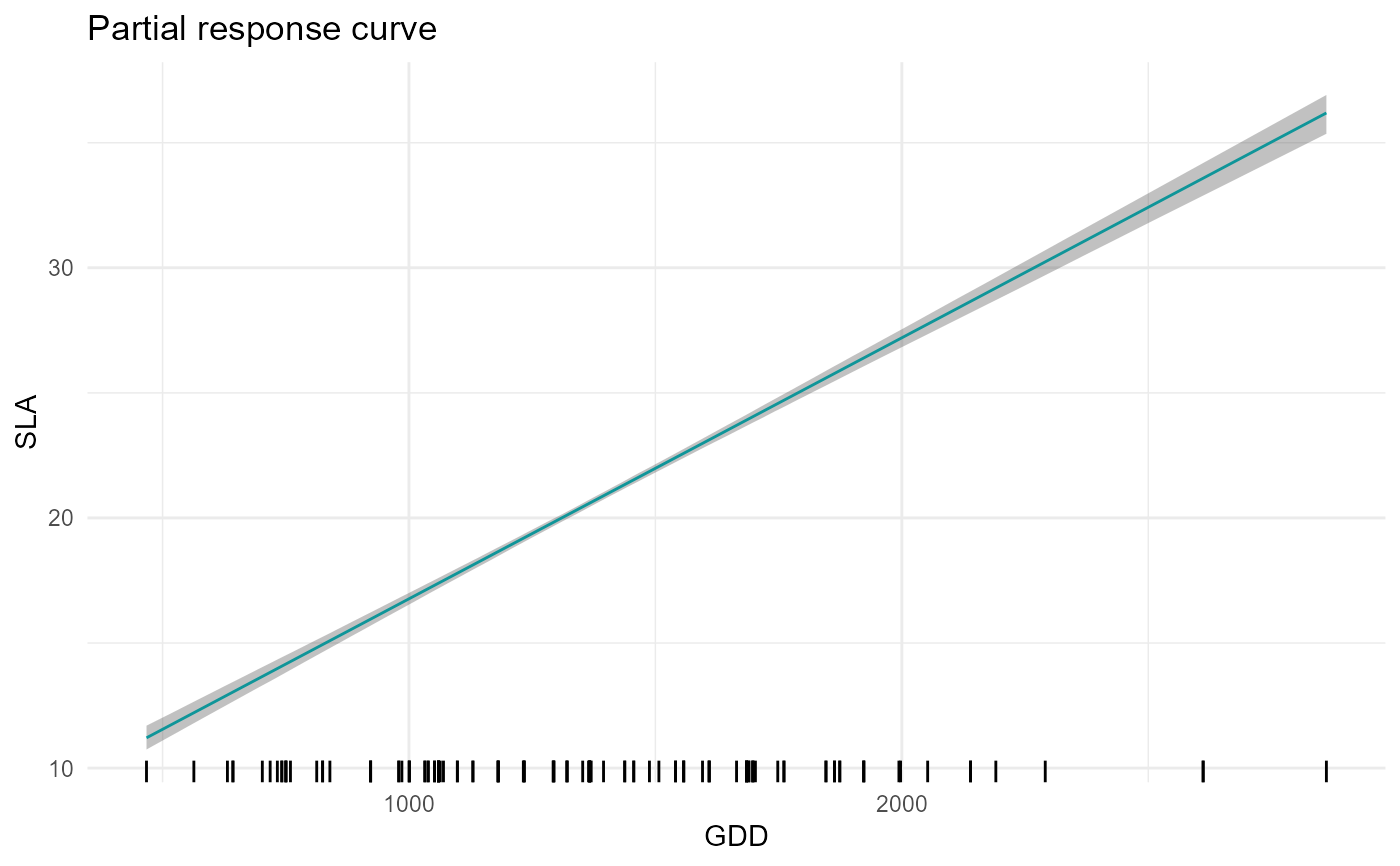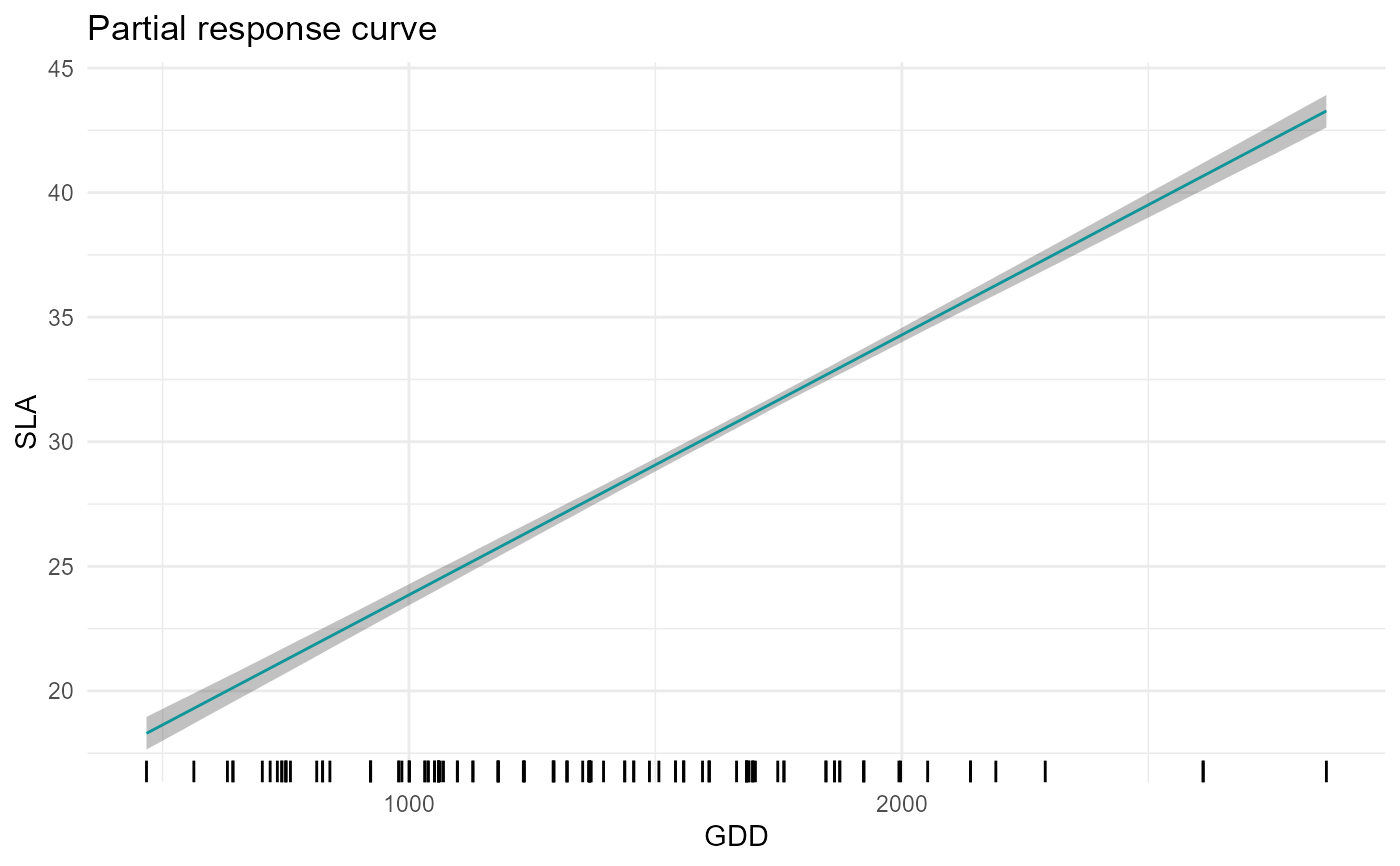Computes and plots the trait-environment relationship of a given CWM trait and a focal environmental variable. In order to build the response curve, the function builds a dataframe where the focal environmental variable varies along a gradient and the other (non-focal) variables are fixed to their mean (but see FixX parameter for fixing non-focal variables to user-defined values).

partial_response(
m,
indexTrait,
XFocal = NULL,
grid.length = 200,
FixX = NULL,
FullPost = "mean"
)

## Arguments

m

a model fitted with jtdm_fit

The name (as specified in the column names of X) of the focal variable.

indexTrait

The name (as specified in the column names of Y) of the focal trait.

XFocal

Optional. A gradient of the focal variable provided by the user. If provided, the function will used this gradient instead of building a regular one. Default to NULL.

grid.length

The number of points along the gradient of the focal variable. Default to 200.

FixX

Optional. A parameter to specify the value to which non-focal variables are fixed. This can be useful for example if we have some categorical variables (e.g. forest vs meadows) and we want to obtain the partial response curve for a given value of the variable. It has to be a list of the length and names of the columns of X. For example, if the columns of X are "MAT","MAP","Habitat" and we want to fix "Habitat" to 1, then FixX=list(MAT=NULL,MAP=NULL,Habitat=1.). Default to NULL.

FullPost

The type of predictions to be obtain. If FullPost = TRUE, the function returns samples from the predictive distribution. If FullPost="mean", the function computes the posterior distribution of the regression term B%*%X). Default to "mean", here FullPost cannot be FALSE.

## Value

A list containing:

p

A plot of the trait-environment relationship.

predictions

A data frame containing the predicted trait-environmental relationships including the gradient of the focal environmental variable, mean trait predictions and quantiles (can be useful to code customized plot).

## Examples

data(Y)
data(X)
# Short MCMC to obtain a fast example: results are unreliable !
m = jtdm_fit(Y=Y, X=X, formula=as.formula("~GDD+FDD+forest"), sample = 1000)
# SLA-GDD relationship
plot$p# SLA-GDD relationship in forest (i.e. when forest=1) plot = partial_response(m,indexGradient="GDD",indexTrait="SLA", FixX=list(GDD=NULL,FDD=NULL,forest=1)) plot$p